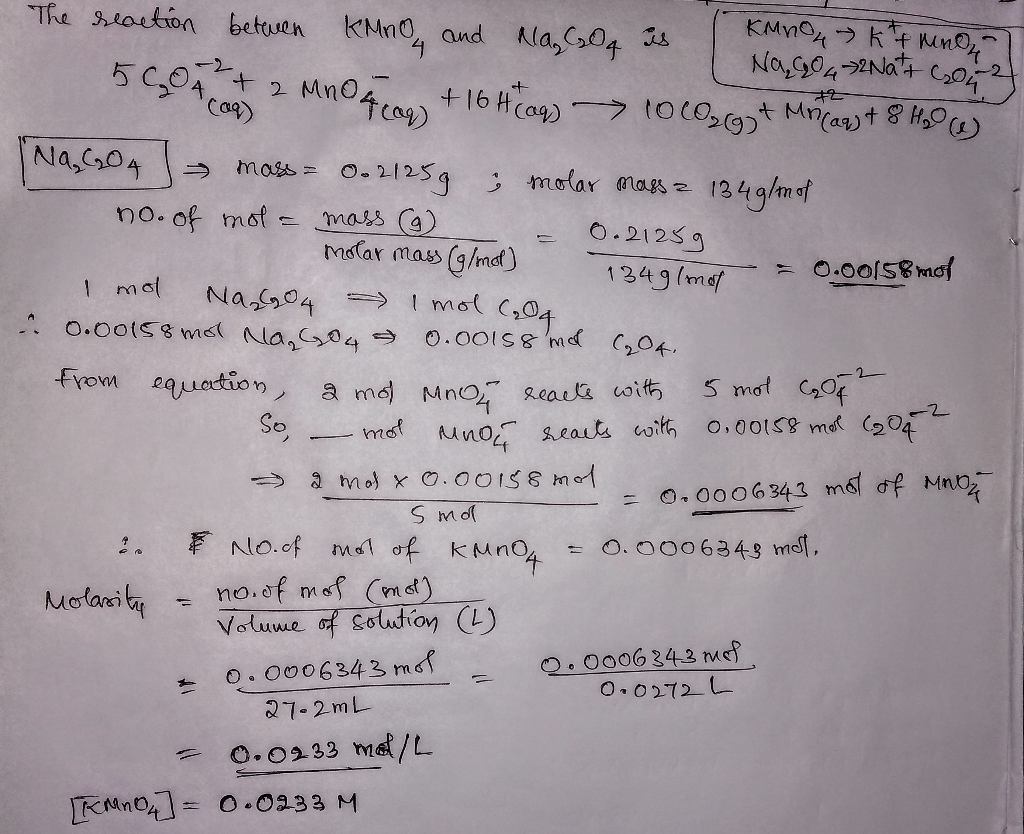I did a redox titration. I need help calculating the concentration of my KMnO4 solution. So i fir...

I did a redox titration. I need help calculating the concentration of my KMnO4 solution. So i first measured .2125 g of Na2C2O4 and put it into a flask. Next I transferred 250 ml of 1 M H2SO4 solution into the flask and dissolved the salt. I then titrated it with KMnO4 with 27.2 ml of KMnO4 solution. What is the concentration of my KMnO4 solution? Please show all the work.thanks and hope you like the answer

Add Answer of: I did a redox titration. I need help calculating the concentration of my KMnO4 solution. So i fir...
More Homework Help Questions Additional questions in this topic.

• How many milliliters of a 0.210 M HI solution are needed to reduce 26.1 mL of a 0.374 M KMnO4...

Need Online Homework Help?